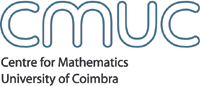Algebra and CombinatoricsMembersSeminarsEventsPublicationsdetails .: download Author(s) Olga Azenhas; Ricardo Mamede; TitleMatrix realization of a pair of tableaux with key and shuffling condition AbstractGiven a pair of tableaux (T,K(\sigma)), where T is a skew-tableau in the alphabet [t] and K(\sigma) is the key associated with \sigma\in St, with the same evaluation as T, we consider the problem of a matrix realization for (T,K(\sigma)) over a local principal ideal domain [1, 2, 3, 4, 5, 6]. It has been shown that the pair (T,K(\sigma)) has a matrix realization only if the word of T is in the plactic class of K(\sigma) . This condition has also been proved suffcient when \sigma is the identity [1, 2, 4], the reverse permutation in St [2, 3], or any permutation in S3 . In each of these cases, the plactic class of K(\sigma) may be described by shuffling together their columns. For t\geq 4 this is no longer true for an arbitrary permutation, but shuffling together the columns of a key always leads to a congruent word. In  A. Lascoux and M. P. Schutzenberger have introduced the notions of frank word and key. It is a simple derivation on Greene's theorem  that words congruent with a key, and frank words are dual of each other as biwords. In this paper, we exhibit, for any \sigma\in St, a matrix realization for the pair (T,K(\sigma)), when the word of T is a shuffle of the columns of K(\sigma). This construction is based on a biword defined by the columns of the key and the places of their letters in the skew-tableau T. The places of these letters are row words which are shuffle components of a frank word. Preprint seriesPré-publicações do Departamento de Matemática da Universidade de Coimbra Issue05-25 Year2005 CMUC Apartado 3008, 3001 - 454 Coimbra, Portugal T:+351 239 791 150 F:+351 239 793 069 cmuc@mat.uc.pt - developed by Flor de Utopia